Courses

# Digital Communications - MCQ Test 2

## 20 Questions MCQ Test GATE ECE (Electronics) 2022 Mock Test Series | Digital Communications - MCQ Test 2

Description
This mock test of Digital Communications - MCQ Test 2 for Electronics and Communication Engineering (ECE) helps you for every Electronics and Communication Engineering (ECE) entrance exam. This contains 20 Multiple Choice Questions for Electronics and Communication Engineering (ECE) Digital Communications - MCQ Test 2 (mcq) to study with solutions a complete question bank. The solved questions answers in this Digital Communications - MCQ Test 2 quiz give you a good mix of easy questions and tough questions. Electronics and Communication Engineering (ECE) students definitely take this Digital Communications - MCQ Test 2 exercise for a better result in the exam. You can find other Digital Communications - MCQ Test 2 extra questions, long questions & short questions for Electronics and Communication Engineering (ECE) on EduRev as well by searching above.
QUESTION: 1

Solution:
QUESTION: 2

### X is a binary memory less source with p (x = 0) = 0.3. If the source is transmitted over a BSC with cross-over probability = 0.1, then the capacity of the channel is

Solution:

Capacity of the channel is

C = 1 -H(0.1)=1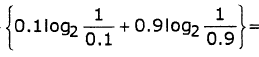= 1 -0.469 = 0.531

QUESTION: 3

### Two binary random variables X and Y are distributed according to the joint distributions p(x = y = 0) = p(x = 0, y = 1) = p(x = y = 1) = 1/3 . Then the entropy H(x) is

Solution:

The marginal probabilities are given by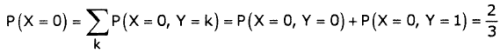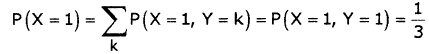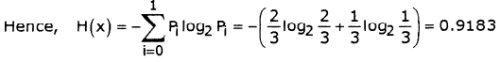*Answer can only contain numeric values
QUESTION: 4

In a Binary digital communication system, the threshold voltage of comparator is 'x' volts and voltage at input of comparator is uniformly distributed in the range of x-0.5 to x+0.25 when '0' is transmitted and it is in the range x-0.25 to x+0.5 when bit '1' is transmitted, the average probability of error in the system is_____________

Solution:

Probability density function of f (z/0) is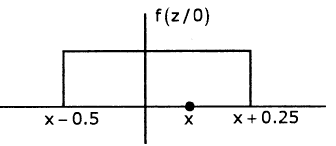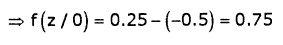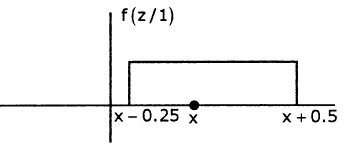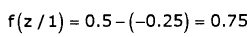When '0' is transmitted, it will be detected as '1' with probability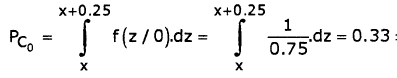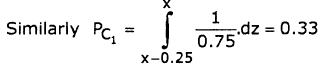Average probability of error = 0.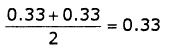QUESTION: 5

If probability of error of PSK signal is 'x', then what will be probability of error of FSK and ASK signal. (Assume x =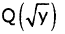Solution: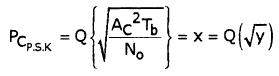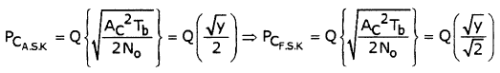As Q-function is not linear operator, we cannot find the probability errors of FSK and ASK with probability error of PSK

QUESTION: 6

If input to matched filter is s(t) = f(t)+ 2f (t)δ(t - 0.5) + f(t - 2.5)*8δ(t+ 0.5)

where f(t) is shown below, then determine the impulse response of matched filter h(t) ; 2 5 ≤ t < 3

(Note: δ is Delta function and * is convolution operator)

Solution: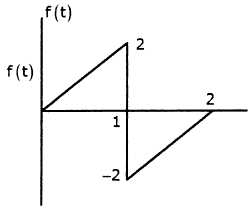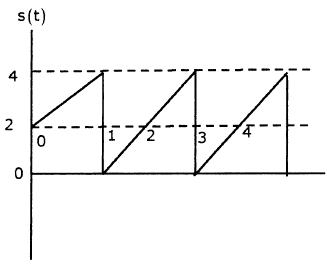2f (t)6(t - 0.5) = 2f(0.5) = 2x1 = 2 f(t-2.5)*6(t+ 0.5) = f(t-(2.5- 0.5))

=f(t-2)

s(t)=f(t)+f(t-2)+2

= -2f +6 ; 2 5 t 5 3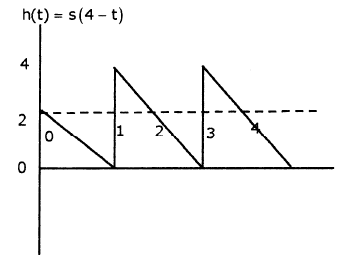QUESTION: 7

Match the following:

Modulation             Type of signaling format to be

technique                     used for modulation

1. ASK               (a)       ON-OFF

2. PSK                (b)       Non return to zero

3. FSK                (c)       Return to zero

4. DPSK

5. QPSK

Solution:

A.S.K signal will be present when 11' is transmitted and absent when '0' is transmitted hence ON -

OFF

In P.S.K for phase reversal a non return to zero format will be used

In FSK

fi (t) = fc (t)+ kfAv             for '1'

fc (t) kfAv              for '0'

hence non return to zero

In D.P.S.K the output of differential enconder will be in ON - OFF format which is than modified using Amplitude level shifting to get NRZ format

In Q.P.S.K

For '10'

Ac cos 27tfct - Ac si n 2/rfct . Hence NRZ

*Answer can only contain numeric values
QUESTION: 8

Consider a BSC with cross-over probability of error P. Suppose that R is the number of bits in a source code word that represents one of 2R possible levels at the output of quantizer. If R = 5, P = 0.01, then the probability of having 5 or less bits in error in a code word is_____________

Solution:

P(5 or less errors in 5 bits) =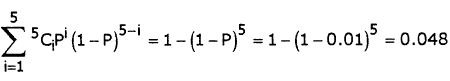*Answer can only contain numeric values
QUESTION: 9

A black and white television picture may be viewed as consisting of approximately 3x105 elements, each of which may occur one of 10 distinct brightness levels with equal probability. Transmission rate is 30 picture frames/ second and SNR is 30 dB. Then the minimum bandwidth required to support the transmission of the resulting video signal is   MHz

Solution:

The information in each element is log210 bits. The information in each picture is [31og210]x105 bits. The transmitted information rate is

[9 log210] x106bits / second.

The channel must have this capacity. From the information capacity theorem,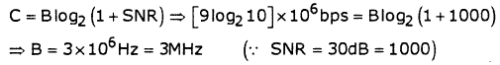QUESTION: 10

A random variable X has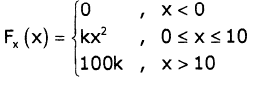Q. The value of constant K is

Solution:

fx(x) = 2kx ; 0 ≤ x ≤ 10  ⇒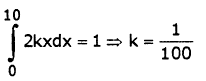QUESTION: 11

A random variable X hasQ. The value of P(4 < x 5 ≤ 8) is

Solution: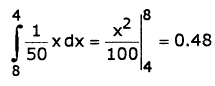QUESTION: 12

A telegraphic source has two symbols dot and dash. The dot duration is 0.2sec. The dash is twice as long as the dot and half as probable.

Q. The entropy of source is

Solution:

P(Dot) + P(Dash) =1

P(Dot)+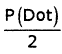= 1

P(Dot) = 2/3P(Dash) = 1/3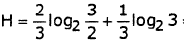= 0.92 bits / symbol

QUESTION: 13

A telegraphic source has two symbols dot and dash. The dot duration is 0.2sec. The dash is twice as long as the dot and half as probable.

Q. The information rate is

Solution:

Number of symbols / sec =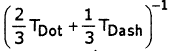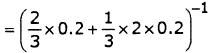= 3.75

Information rate = 3.75 x0.92= 3.45bits / sec

QUESTION: 14

Consider a cascaded block of an LTI system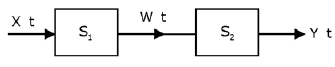The impulse response of S1. is h1. t = δ t -2 The impulse response of 52 is h2 t = t.e-ru t

The input x(t) is wide sense stationary random process with Rxx 0 = 3 and variance = 1

Q. The mean of the random process w(t) is

Solution: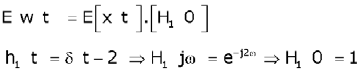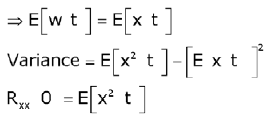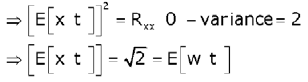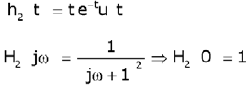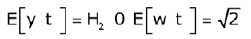QUESTION: 15

Consider a cascaded block of an LTI systemThe impulse response of S1. is h1. t = δ t -2 The impulse response of 52 is h2 t = t.e-ru t

The input x(t) is wide sense stationary random process with Rxx 0 = 3 and variance = 1

Q. If w(t) is input to system 52, then mean of process Y(t) will be

Solution: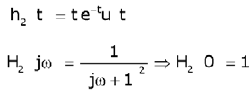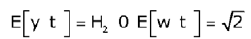QUESTION: 16

CDF of a random variable x is given below.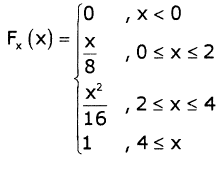Q. E[x] is

Solution: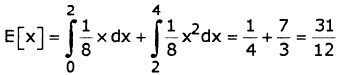Find PDF from CDF, PDF =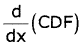QUESTION: 17

CDF of a random variable x is given below.Q. Variance is

Solution: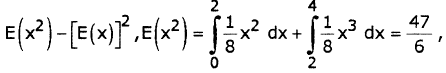Variance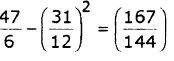QUESTION: 18

Consider a discrete memory less source X with two symbols x1 and x2 and P (xi) = 0.9, P (x2) = 0.1. These symbols are encoded as below.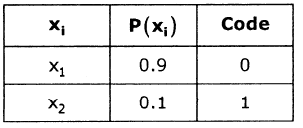Q. Find the source efficiency η

Solution:

average code length L per symbol is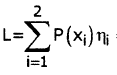= (0.9)1 + (0.1)1 = 1bit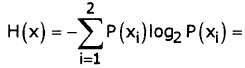-0.9 log2 0.9 - 0.1log2 0.1 = 0.469bits / symbol

code efficiency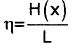= 0.469 = 46.9%

QUESTION: 19

Consider a discrete memory less source X with two symbols x1 and x2 and P (xi) = 0.9, P (x2) = 0.1. These symbols are encoded as below.Q. Find the redundancy γ

Solution:

redundancy r = 1- η =1- 0.469 = 0.531 = 53.1%

QUESTION: 20

The input to a linear delta modulator having a step-size D = 0.628 is a sine wave with frequency fm and peak amplitude Em. If the sampling frequency fs = 40kHz, the combination of the sine-wave frequency and peak amp, where slope overload will take place is

Em                   fin                                         Em                    fin

(a)           0.3V               8kHz                            (b)       1.5V                 4kHz

(c)          1.5V               2kHz                            (d)       3.0V                 1kHz

Solution: